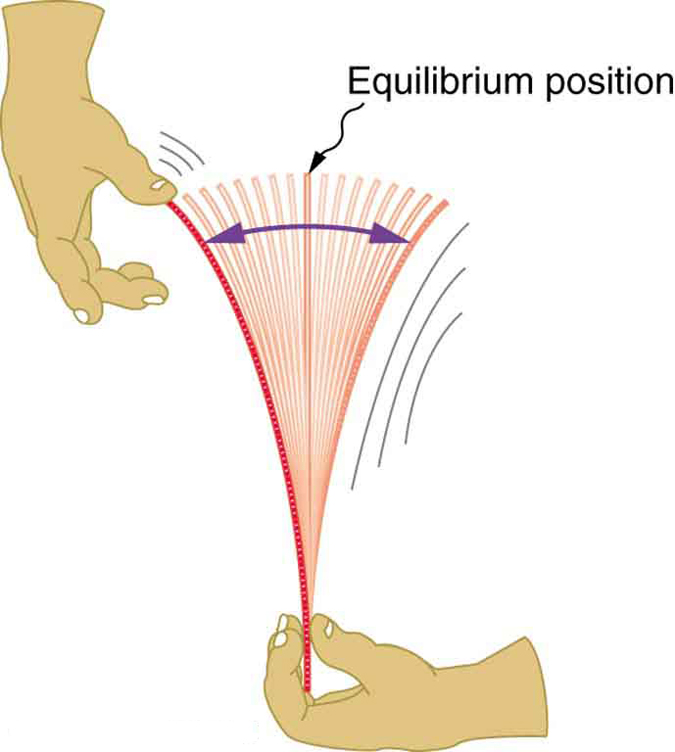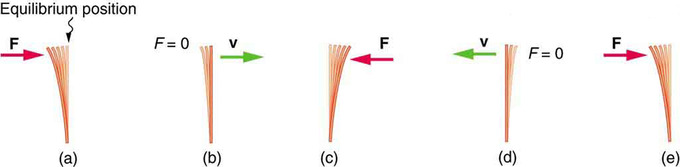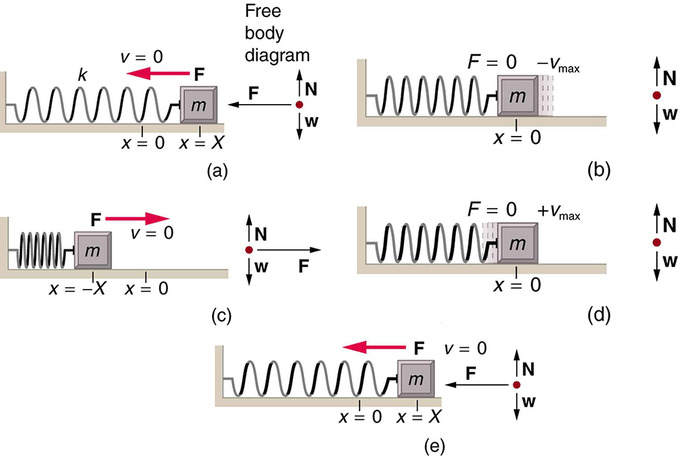# Periodic Motion

• If an object is vibrating to the right and left, then it must have a leftward force on it when it is on the right side, and a rightward force when it is on the left side.
• The restoring force causes an oscillating object to move back toward its stable equilibrium position, where the net force on it is zero.
• The simplest oscillations occur when the restoring force is directly proportional to displacement. In this case the force can be calculated as F=-kx, where F is the restoring force, k is the force constant, and x is the displacement.
• The motion of a mass on a spring can be described as Simple Harmonic Motion(SHM): oscillatory motion that follows Hooke’s Law.
• The period of a mass on a spring is given by the equation  $\text{T}=2\pi \sqrt{\frac{\text{m}}{\text{k}}}$

#### Key Terms

• Restoring force: A variable force that gives rise to an equilibrium in a physical system. If the system is perturbed away from the equilibrium, the restoring force will tend to bring the system back toward equilibrium. The restoring force is a function only of position of the mass or particle. It is always directed back toward the equilibrium position of the system
• amplitude: The maximum absolute value of some quantity that varies.

### Understanding the Restoring Force

Newton’s first law implies that an object oscillating back and forth is experiencing forces. Without force, the object would move in a straight line at a constant speed rather than oscillate. It is important to understand how the force on the object depends on the object’s position. If an object is vibrating to the right and left, then it must have a leftward force on it when it is on the right side, and a rightward force when it is on the left side. In one dimension, we can represent the direction of the force using a positive or negative sign, and since the force changes from positive to negative there must be a point in the middle where the force is zero. This is the equilibrium point, where the object would stay at rest if it was released at rest. It is common convention to define the origin of our coordinate system so that xequals zero at equilibrium.Oscillating Ruler: When displaced from its vertical equilibrium position, this plastic ruler oscillates back and forth because of the restoring force opposing displacement. When the ruler is on the left, there is a force to the right, and vice versa.

Consider, for example, plucking a plastic ruler shown in the first figure. The deformation of the ruler creates a force in the opposite direction, known as a restoring force. Once released, the restoring force causes the ruler to move back toward its stable equilibrium position, where the net force on it is zero. However, by the time the ruler gets there, it gains momentum and continues to move to the right, producing the opposite deformation. It is then forced to the left, back through equilibrium, and the process is repeated until dissipative forces (e.g., friction) dampen the motion. These forces remove mechanical energy from the system, gradually reducing the motion until the ruler comes to rest.Restoring force, momentum, and equilibrium: (a) The plastic ruler has been released, and the restoring force is returning the ruler to its equilibrium position. (b) The net force is zero at the equilibrium position, but the ruler has momentum and continues to move to the right. (c) The restoring force is in the opposite direction. It stops the ruler and moves it back toward equilibrium again. (d) Now the ruler has momentum to the left. (e) In the absence of damping (caused by frictional forces), the ruler reaches its original position. From there, the motion will repeat itself.

### Hooke’s Law

The simplest oscillations occur when the restoring force is directly proportional to displacement. The name that was given to this relationship between force and displacement is Hooke’s law:

$\text{F}=\text{kx}$

Here, F is the restoring force, x is the displacement from equilibrium or deformation, and k is a constant related to the difficulty in deforming the system (often called the spring constant or force constant). Remember that the minus sign indicates the restoring force is in the direction opposite to the displacement. The force constant k is related to the rigidity (or stiffness) of a system—the larger the force constant, the greater the restoring force, and the stiffer the system. The units of k are newtons per meter (N/m). For example, k is directly related to Young’s modulus when we stretch a string. A typical physics laboratory exercise is to measure restoring forces created by springs, determine if they follow Hooke’s law, and calculate their force constants if they do.

Mass on a Spring

A common example of an objecting oscillating back and forth according to a restoring force directly proportional to the displacement from equilibrium (i.e., following Hooke’s Law) is the case of a mass on the end of an ideal spring, where “ideal” means that no messy real-world variables interfere with the imagined outcome.

The motion of a mass on a spring can be described as Simple Harmonic Motion (SHM), the name given to oscillatory motion for a system where the net force can be described by Hooke’s law. We can now determine how to calculate the period and frequency of an oscillating mass on the end of an ideal spring. The period T can be calculated knowing only the mass, m, and the force constant, k:

$\text{T}=2\pi \sqrt{\frac{\text{m}}{\text{k}}}$

When dealing with $\text{f}=1/\text{T}$, the frequency is given by:

$\text{f}=\frac{1}{2\pi }\sqrt{\frac{\text{k}}{\text{m}}}$

We can understand the dependence of these equations on m and k intuitively. If one were to increase the mass on an oscillating spring system with a given k, the increased mass will provide more inertia, causing the acceleration due to the restoring force F to decrease (recall Newton’s Second Law: $\text{F}=\text{ma}$). This will lengthen the oscillation period and decrease the frequency. In contrast, increasing the force constant k will increase the restoring force according to Hooke’s Law, in turn causing the acceleration at each displacement point to also increase. This reduces the period and increases the frequency. The maximum displacement from equilibrium is known as the amplitude X.Motion of a mass on an ideal spring: An object attached to a spring sliding on a frictionless surface is an uncomplicated simple harmonic oscillator. When displaced from equilibrium, the object performs simple harmonic motion that has an amplitude X and a period T. The object’s maximum speed occurs as it passes through equilibrium. The stiffer the spring is, the smaller the period T. The greater the mass of the object is, the greater the period T. (a) The mass has achieved its greatest displacement X to the right and now the restoring force to the left is at its maximum magnitude. (b) The restoring force has moved the mass back to its equilibrium point and is now equal to zero, but the leftward velocity is at its maximum. (c) The mass’s momentum has carried it to its maximum displacement to the right. The restoring force is now to the right, equal in magnitude and opposite in direction compared to (a). (d) The equilibrium point is reach again, this time with momentum to the right. (e) The cycle repeats.

## Simple Harmonic Motion

Simple harmonic motion is a type of periodic motion where the restoring force is directly proportional to the displacement.

### LEARNING OBJECTIVES

Relate the restoring force and the displacement during the simple harmonic motion

### KEY TAKEAWAYS

#### Key Points

• Simple harmonic motion is often modeled with the example of a mass on a spring, where the restoring force obey’s Hooke’s Law and is directly proportional to the displacement of an object from its equilibrium position.
• Any system that obeys simple harmonic motion is known as a simple harmonic oscillator.
• The equation of motion that describes simple harmonic motion can be obtained by combining Newton’s Second Law and Hooke’s Law into a second-order linear ordinary differential equation: ${\text{F}}_{\text{net}}=\text{m}\frac{{\text{d}}^{2}\text{x}}{{\text{dt}}^{2}}=-\text{kx}$.

#### Key Terms

• simple harmonic oscillator: A device that implements Hooke’s law, such as a mass that is attached to a spring, with the other end of the spring being connected to a rigid support, such as a wall.
• oscillator: A pattern that returns to its original state, in the same orientation and position, after a finite number of generations.

### Simple Harmonic Motion

Simple harmonic motion is a type of periodic motion where the restoring force is directly proportional to the displacement (i.e., it follows Hooke’s Law). It can serve as a mathematical model of a variety of motions, such as the oscillation of a spring. In addition, other phenomena can be approximated by simple harmonic motion, such as the motion of a simple pendulum, or molecular vibration.

Simple Harmonic Motion: A brief introduction to simple harmonic motion for calculus-based physics students.

Simple harmonic motion is typified by the motion of a mass on a spring when it is subject to the linear elastic restoring force given by Hooke’s Law. A system that follows simple harmonic motion is known as a simple harmonic oscillator.

### Dynamics of Simple Harmonic Oscillation

For one-dimensional simple harmonic motion, the equation of motion (which is a second-order linear ordinary differential equation with constant coefficients) can be obtained by means of Newton’s second law and Hooke’s law.

${\text{F}}_{\text{net}}=\text{m}\frac{{\text{d}}^{2}\text{x}}{{\text{dt}}^{2}}=-\text{kx}$,

where m is the mass of the oscillating body, x is its displacement from the equilibrium position, and k is the spring constant. Therefore:

$\frac{{\text{d}}^{2}\text{x}}{{\text{dt}}^{2}}=-\left(\frac{\text{k}}{\text{m}}\right)\text{x}$.

Solving the differential equation above, a solution which is a sinusoidal function is obtained.

$\text{x}\left(\text{t}\right)={\text{c}}_{1}\text{cos}\left(\omega \text{t}\right)+{\text{c}}_{2}\text{sin}\left(\omega \text{t}\right)=\text{Acos}\left(\omega \text{t}-\phi \right)$,

where

$\omega =\sqrt{\frac{\text{k}}{\text{m}}}$,

$\text{A}=\sqrt{{\text{c}}_{1}^{2}+{\text{c}}_{2}^{2}}$,

$tan\phi =\left(\frac{{\text{c}}_{2}}{{\text{c}}_{1}}\right)$.

In the solution, c1 and c2 are two constants determined by the initial conditions, and the origin is set to be the equilibrium position. Each of these constants carries a physical meaning of the motion: A is the amplitude (maximum displacement from the equilibrium position), ω = 2πf is the angular frequency, and φ is the phase.

We can use differential calculus and find the velocity and acceleration as a function of time:

$\text{v}\left(\text{t}\right)=\frac{\text{dx}}{\text{dt}}=-\text{A}\omega \text{sin}\left(\omega \text{t}-\phi \right)$

$\text{a}\left(\text{t}\right)=\frac{{\text{d}}^{2}\text{x}}{{\text{dt}}^{2}}=-\text{A}{\omega }^{2}\text{cos}\left(\omega \text{t}-\phi \right)$.

Acceleration can also be expressed as a function of displacement:

$\text{a}\left(\text{t}\right)=-{\omega }^{2}\text{x}$.

Then since ω = 2πf,

$\text{f}=\frac{1}{2\pi }\sqrt{\frac{\text{k}}{\text{m}}}$.

Recalling that $\text{T}=1/\text{f}$,

$\text{T}=2\pi \sqrt{\frac{\text{m}}{\text{k}}}$.

Using Newton’s Second Law, Hooke’s Law, and some differential Calculus, we were able to derive the period and frequency of the mass oscillating on a spring that we encountered in the last section! Note that the period and frequency are completely independent of the amplitude.